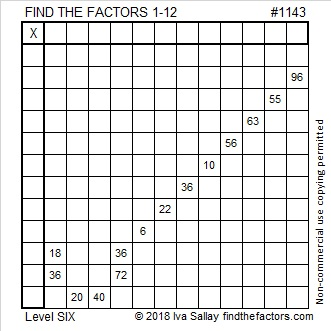# 1143 and Level 6

I hope you get “hooked” on factoring puzzles after you solve this one! It won’t be easy, but if you stick with it, you will be able to solve it. Good luck!Print the puzzles or type the solution in this excel file: 12 factors 1134-1147

Now I’ll share some facts about the number 1143:

• 1143 is a composite number.
• Prime factorization: 1143 = 3 × 3 × 127, which can be written 1143 = 3² × 127
• The exponents in the prime factorization are 2 and 1. Adding one to each and multiplying we get (2 + 1)(1 + 1) = 3 × 2  = 6. Therefore 1143 has exactly 6 factors.
• Factors of 1143: 1, 3, 9, 127, 381, 1143
• Factor pairs: 1143 = 1 × 1143, 3 × 381, or 9 × 127
• Taking the factor pair with the largest square number factor, we get √1143 = (√9)(√127) = 3√127 ≈ 33.808281143 is repdigit 333 in BASE 19 because 3(19² + 19 + 1) = 3(381) = 1143